# Fractions and Decimals Class 7 Notes: Chapter 2

### Introduction: Fractions

The word fraction derives from the Latin word “Fractus” meaning broken. It represents a part of a whole, consisting of a number of equal parts out of a whole.
E.g : slices of a pizza.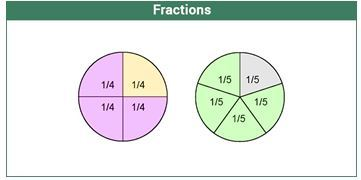#### For more information on Introduction to Fractions, watch the below video.To know more about Fractions, visit here.

### Representation of Fractions

A fraction is represented by 2 numbers on top of each other, separated by a line. The number on top is the numerator and the number below is the denominator. Example :34  which basically means 3 parts out of 4 equal divisions.

#### For more information on Representation of Fractions, watch the below video.### Fractions on the Number Line

In order to represent a fraction on a number line, we divide the line segment between two whole numbers into n equal parts, where n is the denominator.
Example: To represent 1/5 or 3/5, we divide the line between 0 and 1 in 5 equal parts. Then the numerator gives the number of divisions to mark.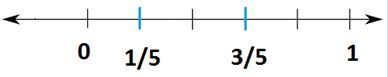To know more about Fractions on the Number Line, visit here.

## Multiplication of Fractions

### Multiplication of Fractions

Multiplication of a fraction by a whole number :
Example 1: 7×(1/3) = 7/3
Example 2 : 5×(7/45) 35/45, Dividing numerator and denominator by 5, we get 7/9

Multiplication of a fraction by a fraction is basically product of numerators/product of denominators

Example 1: (3/5) × (12/13) = 36/65
Example 2 : Multiplication of mixed fractions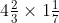First convert mixed fractions to improper fractions and then multiply

$$\begin{array}{l}\frac{14}{3}\times \frac{8}{7}\end{array}$$

### Fraction as an Operator ‘Of’

The ‘of’ operator basically implies multiplication.

Example: 1/6 of 18 = (1/6)×18 = 18/6 3
or, 1/2 of 11 = (1/2) × 11 = 11/2
To know more about Multiplication of Fractions, visit here.

## Division of Fractions

### Reciprocal of a Fraction

Reciprocal of any number n is written as1n
Reciprocal of a fraction is obtained by interchanging the numerator and denominator.
Example: Reciprocal of 2/5 is 5/2
Although zero divided by any number means zero itself, we cannot find reciprocals for them, as a number divided by 0 is undefined.
Example : Reciprocal of 0/7  7/0

### Division of Fractions

Division of a whole number by a fraction : we multiply the whole number with the reciprocal of the fraction.
Example: 63÷(7/5) = 63×(5/7) = 9×45

Division of a fraction by a whole number: we multiply the fraction with the reciprocal of the whole number.
Example: (8/11)÷= (8/11)×(1/4) = 2/11

Division of a fraction by another fraction : We multiply the dividend with the reciprocal of the divisor.
Example: (2/7) ÷ (5/21) = (2/7) × (21/5) = 6/5
To know more about Reciprocal and Division of Fractions, visit here.

## Types of Fractions

### Types of Fractions

Proper fractions represent a part of a whole. The numerator is smaller than the denominator.
Example: 1/4, 7/9, 50/51. Proper fractions are greater than 0 and less than 1

Improper fractions have a numerator that is greater than or equal to the denominator.
Example: 45/6, 6/5. Improper fractions are greater than 1 or equal to 1.

Mixed fractions are a combination of a whole number and a proper fraction.
Example: 43/5 can be written as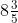.

Conversion of fractions : An improper fraction can be represented as mixed fraction and  a mixed fraction can represented as improper.
In the above case, if you multiply the denominator 5 with the whole number 8 add the numerator 3 to it, you get back 435

Like fractions : Fractions with the same denominator are called like fractions.
Example: 5/7, 3/7. Here we can compare them as (5/7) > (3/7)

Unlike fractions : Fractions with different denominators are called unlike fractions.
Example: 5/3, 9/2. To compare them, we find the L.C.M of the denominator.
Here the L.C.M is 6 So, (5/3)×(2/2) , (9/2)×(3/3)
⇒ 10/6, 27/6
⇒ 27/6 > 10/7

#### For more information on Types of Fractions, watch the below videos.To know more about “Types of Fractions”, visit here.

## Decimals

### Introduction: Decimal

Decimal numbers are used to represent numbers that are smaller than the unit 1. Decimal number system is also known as base 10 system since each place value is denoted by a power of 10.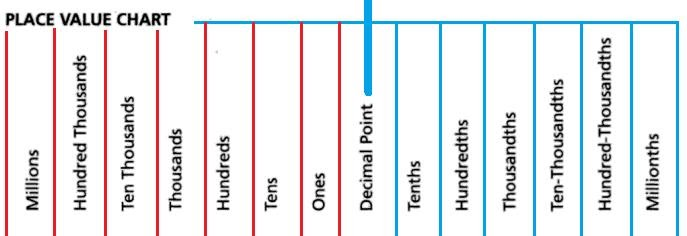A decimal number refers to a number consisting of the following two parts:
(i) Integral part (before the decimal point)
(ii) Fractional Part (after the decimal point).
These both are separated by a decimal separator(.) called the decimal point.

A decimal number is written as follows: Example 564.8 or 23.97.
The numbers to the left of the decimal point increase with the order of 10, while the numbers to the right of the point increase with the decrease order of 10.
The above example 564.8 can be read as ‘five hundred and sixty four and eight tenths’
5×100 6×10 4×8×(1/10)

A fraction can be written as a decimal and vice-versa. Example 3/2 1.5 or 1.5 = 15/10 = 3/2

#### For more information on Fractions and Decimals, watch the below video.To know more about Decimals, visit here.

### Multiplication of Decimals

Multiplication of decimal numbers with whole numbers :
Multiply them as whole numbers. The product will contain the same number of digits after the decimal point as that of the decimal number.
E.g : 11.3×45.2

Multiplication of decimals with powers of 10 :
If a decimal is multiplied by a power of 10, then the decimal point shifts to the right by the number of zeros in its power.
E.g : 45.678×10 456.78 (decimal point shifts by 1 place to the right) or, 45.678×1000 45678 (decimal point shifts by 3 places to the right)

Multiplication of decimals with decimals :

Multiply the decimal numbers without decimal points and then give decimal point in the answer as many places same as the total number of places right to the decimal points in both numbers.

E.g :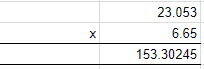### Division of Decimals

Dividing a decimal number by a whole number:
Example: 45.2/55
Step 1. Convert the Decimal number into Fraction: 45.25= 4525/100
Step 2. Divide the fraction by the whole number: (4525/100)÷= (4525/100) × (1/5) 9.05

Dividing a decimal number by a decimal number:
Example 1: 45.25/0.5
Step 1. Convert both the decimal numbers into fractions: 45.25 = 4525/100 and 0.5 = 5/10
Step 2. Divide the fractions: (4525/100)÷(5/10) = (4525/100)×(10/5) = 90.5
Example 2: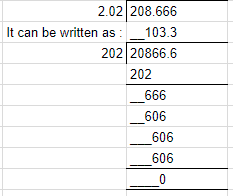Dividing a decimal number by powers of 10  :
If a decimal is divided by a power of 10, then the decimal point shifts to the left by the number of zeros present in the power of 10.
Example: 98.765÷100=0.98765 Infinity

When the denominator in a fraction is very very small (almost tending to 0), then the value of the fraction tends towards infinity.
E.g: 999999/0.000001 = 999999000001  a very large number, which is considered to be

To know more about Multiplication and Division of Decimals, visit here.

## Frequently Asked Questions on CBSE Class 7 Maths Notes Chapter 2 Fractions and Decimals

Q1

### What is a decimal?

A decimal is a fraction written in a special form.

Q2

### What is a decimal fraction?

In algebra, a decimal fraction is a fraction whose denominator is 10 or a multiple of 10 like 100, 1,000, 10,000, etc.

Q3

### What does reciprocal mean?

In Mathematics, reciprocal means an expression which when multiplied by another expression, gives one (1) as the result.